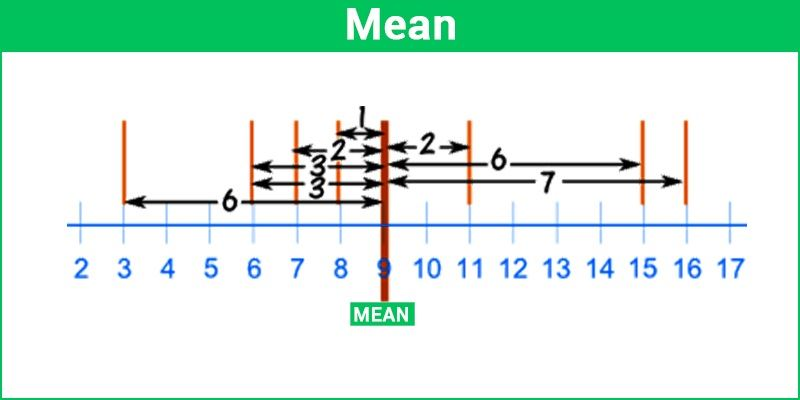# Mean

Mean is an average of the given numbers: a calculated central value of a set of numbers. In simple words, it is the average. It’s also the meanest because it takes the most math to figure it out.  Measures of central tendency, or averages, are used in a variety of contexts and form the basis of statistics. The formula to calculate the mean is ratio of the total of observations to number of observations.

Some students get confused with mean and median in statistics. One should always remember that mean is the average value of any given data set while median is the middle value when the data set is arranged in an order. Let us discuss more mean here.## Definition of Mean in Statistics

Mean is nothing but the average of given set of values. It denotes the equal distribution of values for a given data set. Central tendency is the statistical measure that recognises a single value as representative of the entire distribution. It strives to provide an exact description of the whole data. It is the unique value that is the which represents collected data. The mean, median and mode are the three commonly used measures of central tendency.

In the case of a discrete probability distribution of a random variable X, the mean is equal to the sum over every possible value weighted by the probability of that value; that is, it is computed by taking the product of each possible value x of X and its probability P(x), and then adding all these products together.

## What is Mean in Maths?

Mean, in Maths, is nothing but the average value of the given numbers or data. To calculate the mean, we need to add the total values given in a datasheet and then divide the sum by the total number of values. Suppose, in a data table, the price values of 10 clothing materials are mentioned. If we have to find the mean of the prices, then add the prices of each clothing material and divide the total sum by 10. It will result in average value.

Another example is, if we have to find the average age of students of a class, then we have to add the age of individual student present in the class and then divide the sum by the total number of students present in the class.

Mean is a method which is commonly used in Statistics. In primary schools, we have learned this concept with the term ‘average’. But in higher classes, when we are introduced with the topic mean, it refers to an advanced version of sequence or series of a number. In real-world, when there is huge data available, we use statistics to deal with it. Along with mean, we also learn about median and mode. Median is the middle value of a given data when all the values are arranged in ascending order. Whereas mode is the number in the list, which is repeated a maximum number of times.

## Mean Formula

The basic formula to calculate the mean is calculated based on the given data set. Each term in the data set is considered while evaluating the mean. The general formula for mean is given by the ratio of sum of all the terms and total number of terms. Hence, we can say;

Mean = Sum of the Given Data/Total number of Data

To calculate the arithmetic mean of a set of data we must first add up (sum) all of the data values (x) and then divide the result by the number of values (n). Since ∑ is the symbol used to indicate that values are to be summed (see Sigma Notation) we obtain the following formula for the mean (x̄):

x̄=∑ x/n

## How to Find Mean?

To find the mean of any given data sets, we have to use take the average.

For example, in a class there are 20 students and they have secured a percentage of: 88,82,88,85,84,80,81,82,83,85,84,74,75,76,89,90,89,80,82,83. Find the average of percentage obtained by the class.

Solution: Average = Total of percentage obtained by 20 students in class/Total number of students

Avg = [88+82+88+85+84+80+81+82+83+85+84+74+75+76+89+90+89+80+82+83]/20

Avg.=1660/20 = 83

Hence, the average of percentage got by each student in class is 83%.

In the same, we find the mean of the given data set, in statistics.

## Types of Mean

There are majorly three different types of mean value which you will be studying in statistics.

1. Arithmetic Mean
2. Geometric Mean
3. Harmonic Mean

### Arithmetic Mean

When you add up all the values and divide by the number of values it is called Arithmetic Mean. To calculate, just add up all the given numbers then divide by how many numbers are given.

Example: What is the mean of 3, 5, 9, 5, 7, 2?

Now add up all the given numbers:

3 + 5 + 9 + 5 + 7 + 2 = 31

Now divide by how many numbers provided in the sequence:

316= 5.16

### Geometric Mean

The geometric mean of two numbers x and y is xy. If you have three numbers x, y, and z, their geometric mean is 3xyz.

$\large Geometric\;Mean=\sqrt[n]{x_{1}x_{2}x_{3}…..x_{n}}$

Example: Find the geometric mean of 4 and 3 ?

Geometric Mean =$\sqrt{4 \times 3} = 2 \sqrt{3} = 3.46$

### Harmonic Mean

The harmonic mean is used to average ratios. For two numbers x and y, the harmonic mean is 2xy(x+y). For, three numbers x, y, and z, the harmonic mean is 3xyz(xy+xz+yz)

$\large Harmonic\;Mean (H) = \frac{n}{\frac{1}{x_{1}}+\frac{1}{x_{2}}+\frac{1}{x_{2}}+\frac{1}{x_{3}}+……\frac{1}{x_{n}}}$

$\large X_{rms}=\sqrt{\frac{x_{1}^{2}+x_{2}^{2}+x_{3}^{2}….x_{n}^{2}}{n}}$
$\large \frac{(x_{1}^{2}+x_{2}^{2}+….+x_{n}^{2})}{(x_{1}+x_{2}+…..x_{n})}$